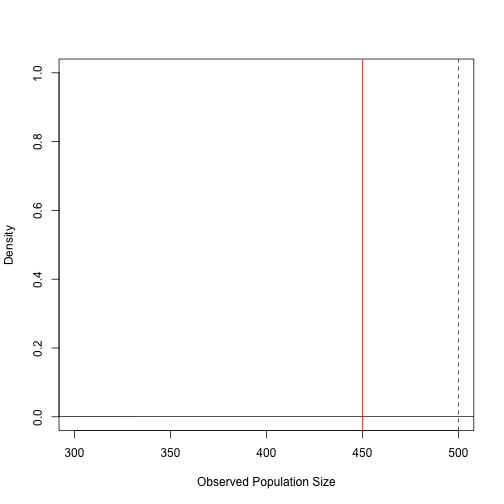We present a small example from “Entity Resolution with Emprically Motivated Priors”, Bayesian Analysis, (10),4:849-975. We will be using the RecordLinkage package in R and the RLdata500 data set.

The blink package removes duplicate entries from multiple databases using the method outlined in the paper above. We illustrate an example of using this package using a German dataset comprised of first and last name and full date of birth.

Our goals include

• Presenting the RLdata500 dataset with summary information.
• Illustrating how we can format the RLdata500 dataset to work with the blink package
• Examples for setting the tuning parameters for blink
• Running the Gibbs sampler in blink
• Illustrating a few plots and sample output

## Understanding the RLdata500 dataset

The RLdata500 dataset exists already in the RecordLinkage package in R. We review this data set for the user.

The RLdata500 data consists of 500 records with 10 percent duplication. Thus, there are 450 unique individuals. There is full information on each record containing first name, last name, and full date of birth.

We first load the Record Linkgae package and load the RLdata500 data set. We also, provide the first few lines of the data.

``````library(RecordLinkage)
data(RLdata500)
``````
``````##   fname_c1 fname_c2 lname_c1 lname_c2   by bm bd
## 1  CARSTEN     <NA>    MEIER     <NA> 1949  7 22
## 2     GERD     <NA>    BAUER     <NA> 1968  7 27
## 3   ROBERT     <NA> HARTMANN     <NA> 1930  4 30
## 4   STEFAN     <NA>    WOLFF     <NA> 1957  9  2
## 5     RALF     <NA>  KRUEGER     <NA> 1966  1 13
## 6  JUERGEN     <NA>   FRANKE     <NA> 1929  7  4
``````

## Preparing the data

Next, we prepare the data for working with the blink package.

``````# X.c contains the categorical variables
# X.s contains the string variables
# p.c is the number of categorical variables
# p.s contains the number of string variables
X.c <- RLdata500[c("by","bm","bd")]
X.c <- as.matrix(RLdata500[,"bd"],ncol=1)
p.c <- ncol(X.c)
X.s <- as.matrix(RLdata500[-c(2,4,7)])
p.s <- ncol(X.s)
``````

## Setting the Tuning Parameters

Now, we give a small example for setting the tuning parameters before running the Gibbs sampler.

First, we work with a file number identifier.

``````# File number identifier
# Note: Recall that X.c and X.s include all files "stacked" on top of each other.
# The vector below keeps track of which rows of X.c and X.s are in which files.
file.num <- rep(c(1,2,3),c(200,150,150))
``````

Next, we work with the parameters that tune the prior on the amount of distortion that goes into the model.

``````# Subjective choices for distortion probability prior
a <-1
b <- 999
``````

Then we write a function for the Edit distance between two strings. Other distance functions could be used, such as Jaro-Winkler.

``````d <- function(string1,string2){adist(string1,string2)}
``````

For the steepness parameter, we recommend

``````c <- 1
``````

## The Gibbs Sampler for bLink

We now run a test version of the Gibbs sampler using blink, with 10 Gibbs iterations and a maximum size of M=500 (assuming the overall known population size is 500).

``````library(knitr)
``````
``````## Loading required package: stringdist
``````
``````## Loading required package: plyr
``````
``````library(plyr)
Sys.setenv(TMPDIR="/tmp/")
configure.vars="TMPDIR=/tmp/"
lam.gs <- rl.gibbs(file.num=file.num,X.s=X.s,X.c=X.c,num.gs=2,a=a,b=b,c=c,d=d, M=500)
#system.time(lam.gs <- rl.gibbs(file.num=file.num,X.s=X.s,X.c=X.c,num.gs=2,a=a,b=b,c=c,d=d, M=500))
``````

``````#estLink <- tempfile(pattern = "lam.gs")
estPopSize <- apply(estLink , 1, function(x) {length(unique(x))})
plot(density(estPopSize),xlim=c(300,500),main="",lty=1, "Observed Population Size", ylim= c(0,1))
abline(v=450,col="red")
abline(v=mean(estPopSize),col="black",lty=2)
mean(estPopSize)
``````
``````##  500
``````
``````sd(estPopSize)
``````
``````##  0
``````For more information, such as how to use the recall, precision, and other summary statistics, please see the paper.